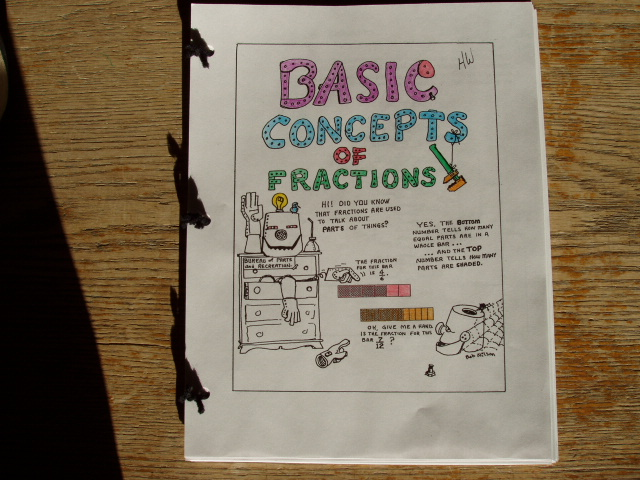Home Grades 5-8 Sample Fraction Bars Worksheets The eight major sections of the Grades 5-8 Fraction Bars Teacher Resource Package have an average of 13 worksheets each. The tops of many worksheets have an explanation or example that connects to the Fraction Bars model from the games and activities in the Teacher's Guide. Each of the eight section begins with a full-page cartoon and students sometimes use these as the cover of a booklet containing the worksheets from a given section (see photo below). At the end of each section there are two pages of word problems for the given topic. A sample worksheet has been selected for each of the following topics: Basic Concepts; Equality; Inequality Addition; Subtraction; Multiplication; Division; and Fractions-Decimals-Percents. Basic ConceptsEquality Inequality Addition Subtraction Multiplication Division Fractions-Decimals-Percents